Learn All Concepts of Chapter 3 Class 12 Matrices - FREE. Check - Matrices Class 12 - Full video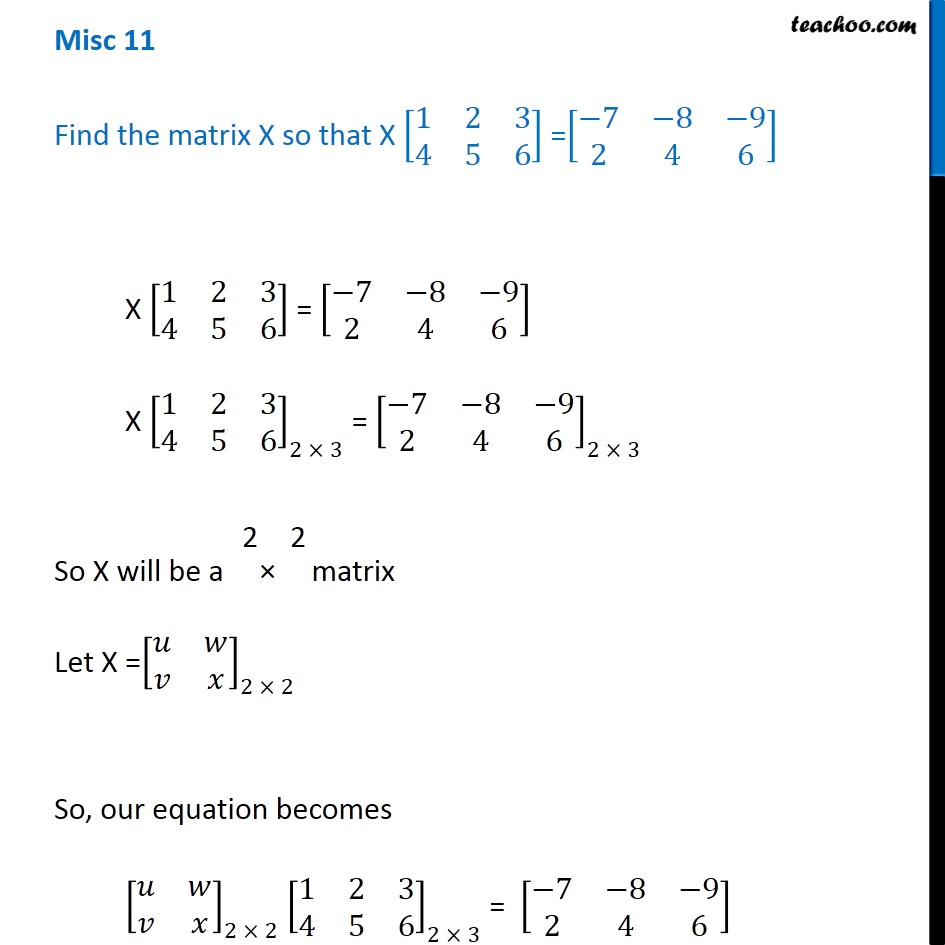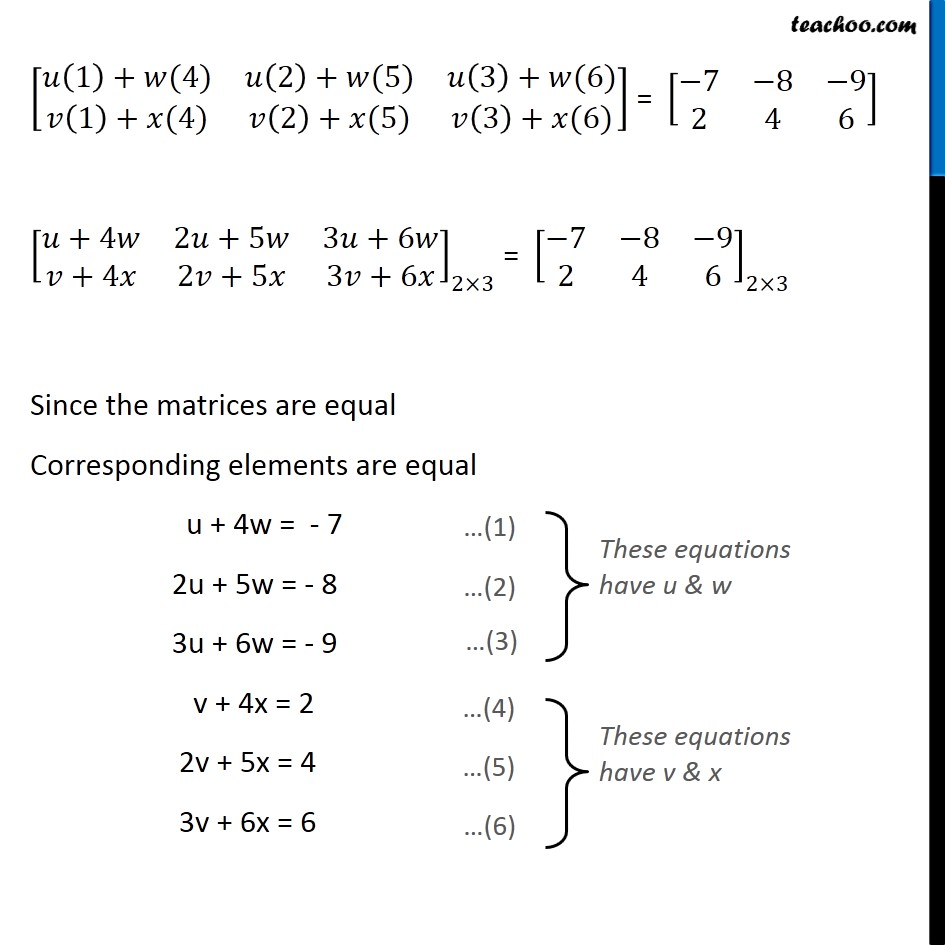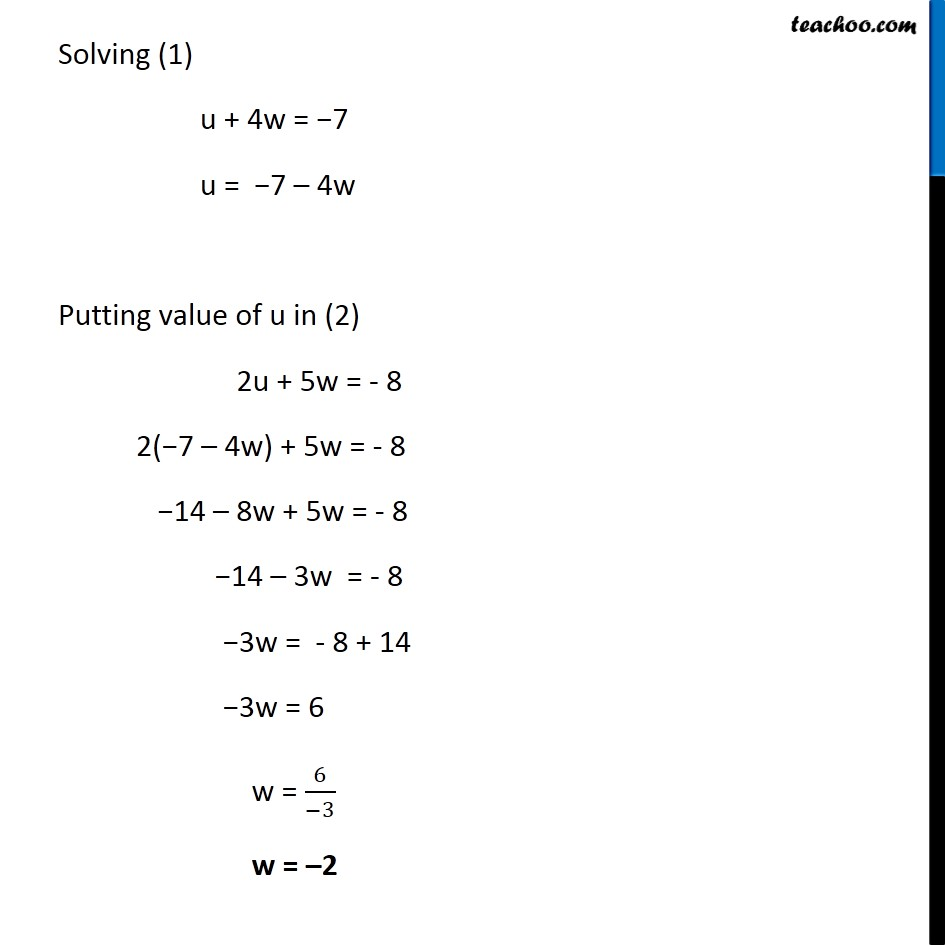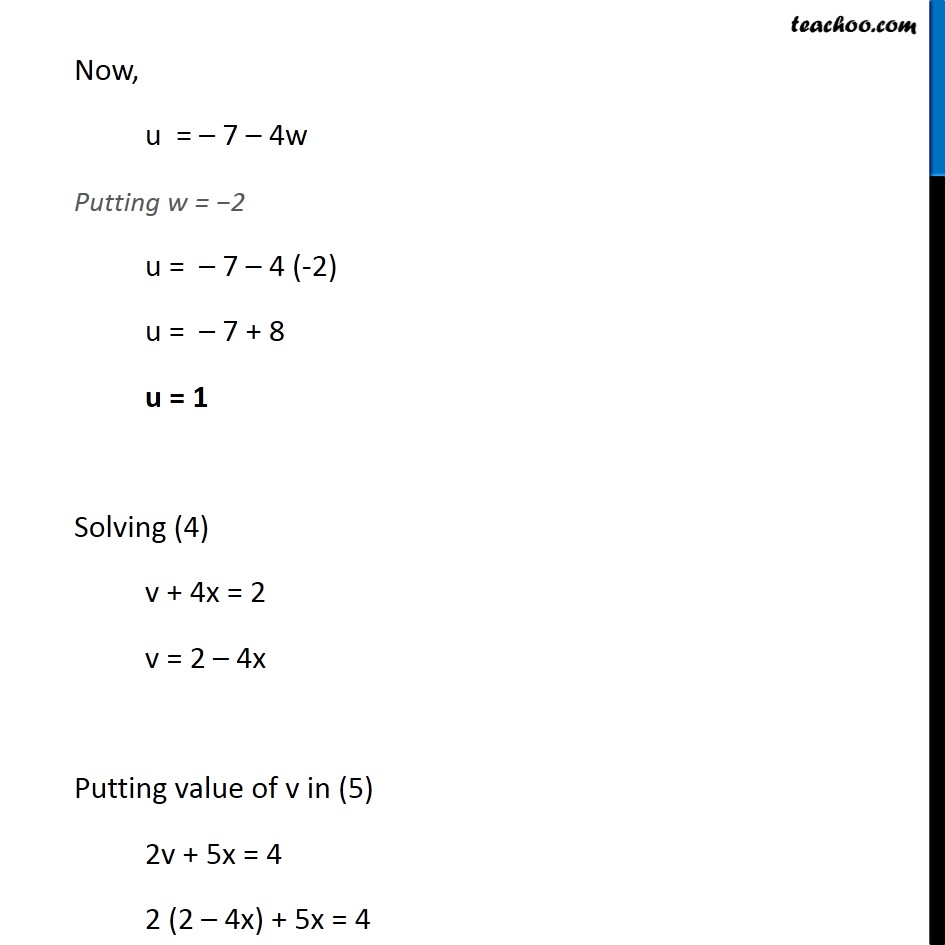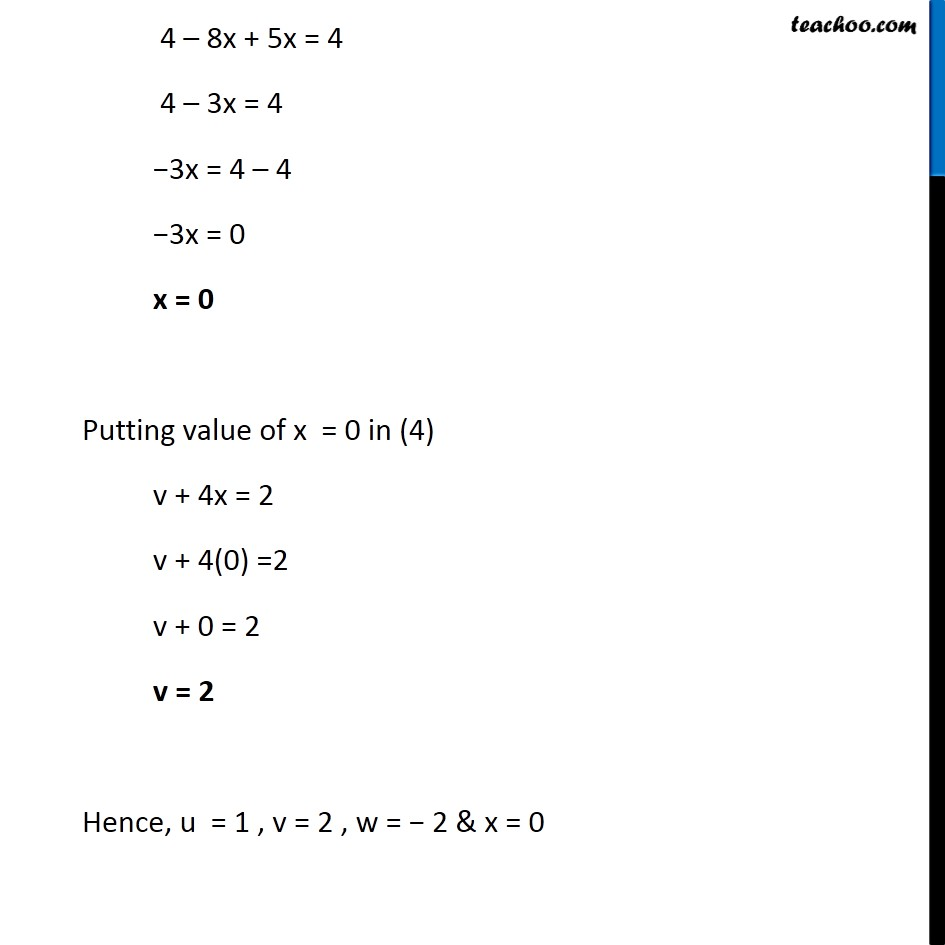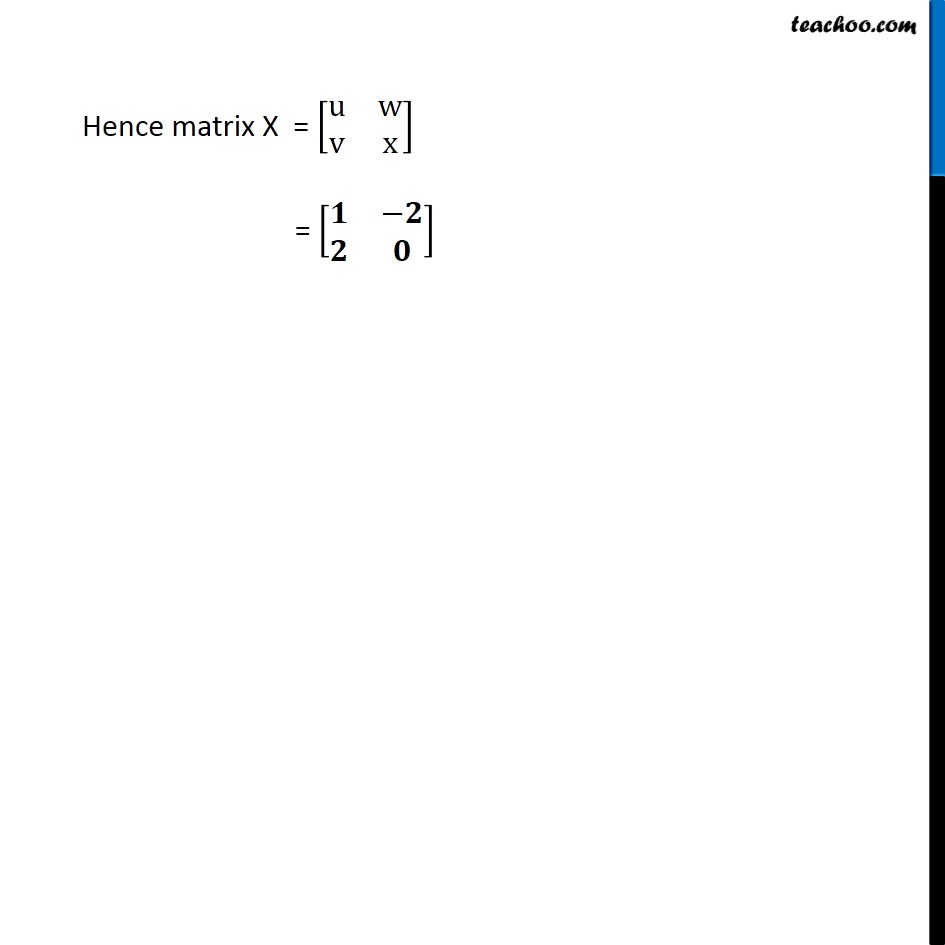1. Chapter 3 Class 12 Matrices
2. Concept wise
3. Finding unknown - Matrice

Transcript

Misc 11 Find the matrix X so that X [■8(1&2&3@4&5&6)] =[■8(−7&−8&−9@2&4&6)] X [■8(1&2&3@4&5&6)] = [■8(−7&−8&−9@2&4&6)] X [■8(1&2&3@4&5&6)]_(2 × 3) = [■8(−7&−8&−9@2&4&6)]_(2 × 3) So X will be a × matrix Let X =[■8(𝑢&𝑤@𝑣&𝑥)]_(2 × 2) So, our equation becomes [■8(𝑢&𝑤@𝑣&𝑥)]_(2 × 2) [■8(1&2&3@4&5&6)]_(2 × 3) = [■8(−7&−8&−9@2&4&6)] [■8(𝑢(1)+𝑤(4)&𝑢(2)+𝑤(5)&𝑢(3)+𝑤(6)@𝑣(1)+𝑥(4)&𝑣(2)+𝑥(5)&𝑣(3)+𝑥(6))] = [■8(−7&−8&−9@2&4&6)] [■8(𝑢+4𝑤&2𝑢+5𝑤&3𝑢+6𝑤@𝑣+4𝑥&2𝑣+5𝑥&3𝑣+6𝑥)]_(2×3) = [■8(−7&−8&−9@2&4&6)]_(2×3) Since the matrices are equal Corresponding elements are equal u + 4w = - 7 2u + 5w = - 8 3u + 6w = - 9 v + 4x = 2 2v + 5x = 4 3v + 6x = 6 These equations have u & w These equations have u & w These equations have u & w Solving (1) u + 4w = −7 u = −7 – 4w Putting value of u in (2) 2u + 5w = - 8 2(−7 – 4w) + 5w = - 8 −14 – 8w + 5w = - 8 −14 – 3w = - 8 −3w = - 8 + 14 −3w = 6 w = 6/(−3) w = –2 Now, u = – 7 – 4w Putting w = −2 u = – 7 – 4 (-2) u = – 7 + 8 u = 1 Solving (4) v + 4x = 2 v = 2 – 4x Putting value of v in (5) 2v + 5x = 4 2 (2 – 4x) + 5x = 4 4 – 8x + 5x = 4 4 – 3x = 4 −3x = 4 – 4 −3x = 0 x = 0 Putting value of x = 0 in (4) v + 4x = 2 v + 4(0) =2 v + 0 = 2 v = 2 Hence, u = 1 , v = 2 , w = − 2 & x = 0 4 – 8x + 5x = 4 4 – 3x = 4 −3x = 4 – 4 −3x = 0 x = 0 Putting value of x = 0 in (4) v + 4x = 2 v + 4(0) =2 v + 0 = 2 v = 2 Hence, u = 1 , v = 2 , w = − 2 & x = 0 Hence matrix X = [■8(u&w@v&x)] = [■8(𝟏&−𝟐@𝟐&𝟎)]

Finding unknown - Matrice